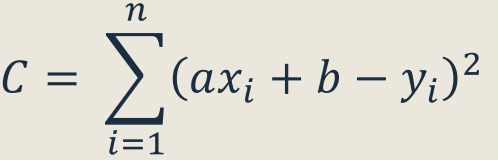top of page
Search

# Computer Vision Assignment Help | Linear Least Squares Optimization

### Introduction

• Main idea: approximating the parameters of a model to given data

• Definition: minimizes the sum of squared differences between the given data and the function value obtained from the modeled parameters

• Applications: regression problems (curve fitting), computer vision (image alignment)

### Linear Least Squares Optimization (LLSO)

Linear refers to the parameters, not the line fitting the data

LLSO for regression fits data using a function of the form:Suppose we are given n data points and want to determine the equation of the line y=mx+b that best fits the data. Then our objective will be set as:where C= the objective function and (xi, yi) = given data points

We need to determine model parameters a and b that minimize the above objective function.

To determine a and b that minimizes C, we take the partial derivatives with respect to each parameter and equate to 0:Example:### Python Implementation

```import sys
import numpy as np
import matplotlib.pyplot as plt
def main():
#d = 2*5.2 + 4 * 6.7 + 6 * 9.1 + 8 * 10.9
#print(d)
#e = 2*5.2 + 2*6.7 +2*9.1 + 2*10.9
#print(e)
x = np.ndarray((4,1))
y = np.ndarray((4,1))
x[0,0] = 1
x[1,0] = 2
x[2,0] = 3
x[3,0] = 4
y[0,0] = 5.2
y[1,0] = 6.7
y[2,0] = 9.1
y[3,0] = 10.9
A = np.ndarray((2,2))
A[0,0] = 60
A[0,1] = 20
A[1,0] = 20
A[1,1] = 8
ainv = np.linalg.inv(A)
z = np.ndarray((2,1))
z[0,0] = 179
z[1,0] = 63.8
res = np.dot(ainv,z) # a = res[0,0] and b=[1,0]
print(res)
# do a scatter plot of the data
area = 10
colors =['black']
plt.scatter(x, y, s=area, c=colors, alpha=0.5, linewidths=8)
plt.title('Linear Least Squares Regression')
plt.xlabel('x')
plt.ylabel('y')
#plot the fitted line
yfitted = x*res[0,0] + res[1,0]
line,=plt.plot(x, yfitted, '--', linewidth=2) #line plot
line.set_color('red')
plt.show()

if __name__ == "__main__":
sys.exit(int(main() or 0))```

Output:

[[1.95]

[3.1 ]]If you are looking to hire computer vision assignment, project, homework then send your assignment requirements details at realcode4you@gmail.com and get instant help with an affordable price.